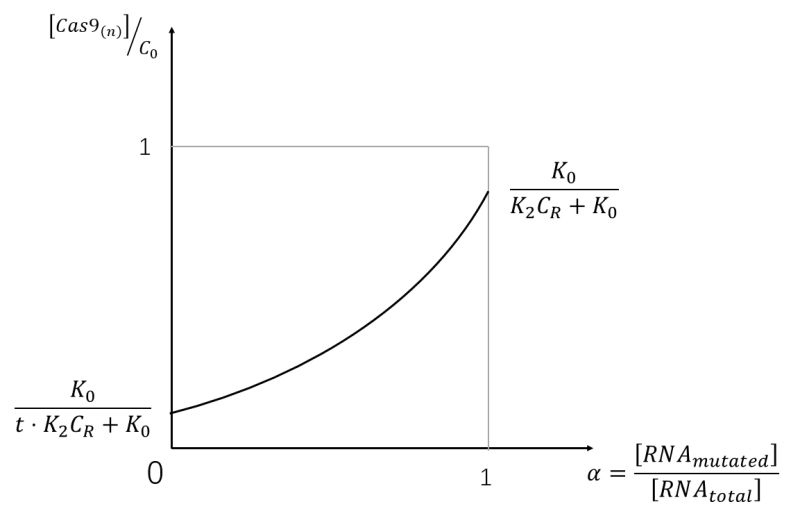Safety

# modeling

We model our system mainly by the following assumptions:
1. PAMmer and sgRNA of the target gene are abundant, so all the suvCas9 is bound with them;
$$suvCas9 + sgRNA + PAMmer \to suvCas9::sgRNA::PAMmer$$
2. The total concentration of suvCas9 (located in cytoplasm and nucleus) and the target mRNA (normal and mutated) stay the same;
$$[suvCas9::sgRNA::PAMmer(cytosolic)] + [suvCas9::sgRNA::PAMmer(nucleic)] + [mRNA(canonical)::suvCas9::sgRNA::PAMmer(cytosolic)] + [mRNA(mutated)::suvCas9::sgRNA::PAMmer(cytosolic)] = {c}_{0}$$ $$mRNA(mutated) + mRNA(canonical) = {c}_{R}$$
3. All the related reactions, which are listed below, obey the steady-state assumption, i. e., they are in the equilibrium state; $$suvCas9::sgRNA::PAMmer(cytosolic) \to suvCas9::sgRNA::PAMmer(nucleic), {K}_{0}$$ $$mRNA(canonical) + suvCas9::sgRNA::PAMmer(cytosolic) \to mRNA(canonical)::suvCas9::sgRNA::PAMmer(cytosolic), {K}_{1}$$ $$mRNA(mutated) + suvCas9::sgRNA::PAMmer(cytosolic) \to mRNA(mutated)::suvCas9::sgRNA::PAMmer(cytosolic), {K}_{2}$$
4. After binding with mRNA, the suvCas9 complex cannot enter the nucleus; Using the above mentioned assumptions, we can know: $$[suvCas9::sgRNA::PAMmer(nucleic)] = \frac{{K}_{0}}{1 + {K}_{0} + {K}_{1}[mRNA(canonical)] + {K}_{2}[mRNA(mutated)]} \times {c}_{0}......(1)$$
We also assume: $$[mRNA(mutated)] = \alpha \times {c}_{R}$$ $$[mRNA(canonical)] = (1- \alpha) \times {c}_{R}$$ $${K}_{1} = t \times {K}_{2}, t >> 1$$ In fact: $${K}_{0} >> 1$$ So, the equation (1) can be modified to: $$[suvCas9::sgRNA::PAMmer(nucleic)] / {c}_{0} = \frac{{K}_{0}}{{K}_{2}{c}_{R}(1 - t)\alpha + {K}_{2}{c}_{R}t + {K}_{0}}......(2)$$ The equation (2) is plotted below:The relation between suvCas9::sgRNA::PAMmer(nucleic) and thymidine kinase(TK) expression is linear in the working condition: $$[TK] = {k}_{0}[suvCas9::sgRNA::PAMmer(nucleic)]......(3)$$ We assume a sigmoid function between [TK] and probability of cell death: $$P(cell death) = \frac{1}{1 + e^{-{k}_{1}[TK] + {k}_{2}}}......(4)$$ Integrating the equation (2), (3) and (4), we get: $$P(cell death) = \frac{1}{1 + e^{{k}_{2} - {c}_{0}{k}_{0}{k}_{1}\frac{{K}_{0}}{{K}_{2}{c}_{R}(1 - t)\alpha + {K}_{2}{c}_{R}t + {K}_{0}}}}$$ It can also be written in the logarithm relative risk format: $$log(\frac{P(cell death)}{1 - P(cell death)}) = {c}_{0}{k}_{0}{k}_{1}\frac{{K}_{0}}{{K}_{2}{c}_{R}(1 - t)\alpha + {K}_{2}{c}_{R}t + {K}_{0}} - {k}_{2}$$ In this final equation, we can modify several parameters: ${c}_{0}, {k}_{0}$ by the following method: ${c}_{0}$: By changing the suvCas9 promotor and terminator. ${k}_{0}$: By changing the inducible promotor of the TK gene